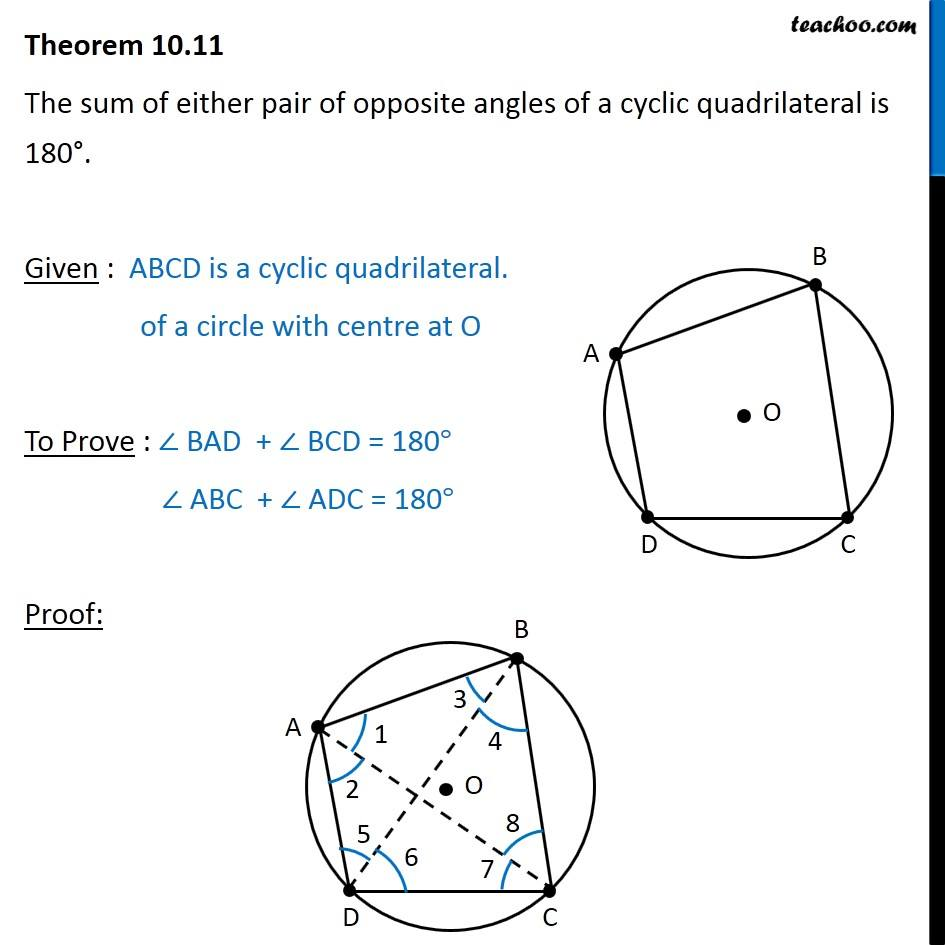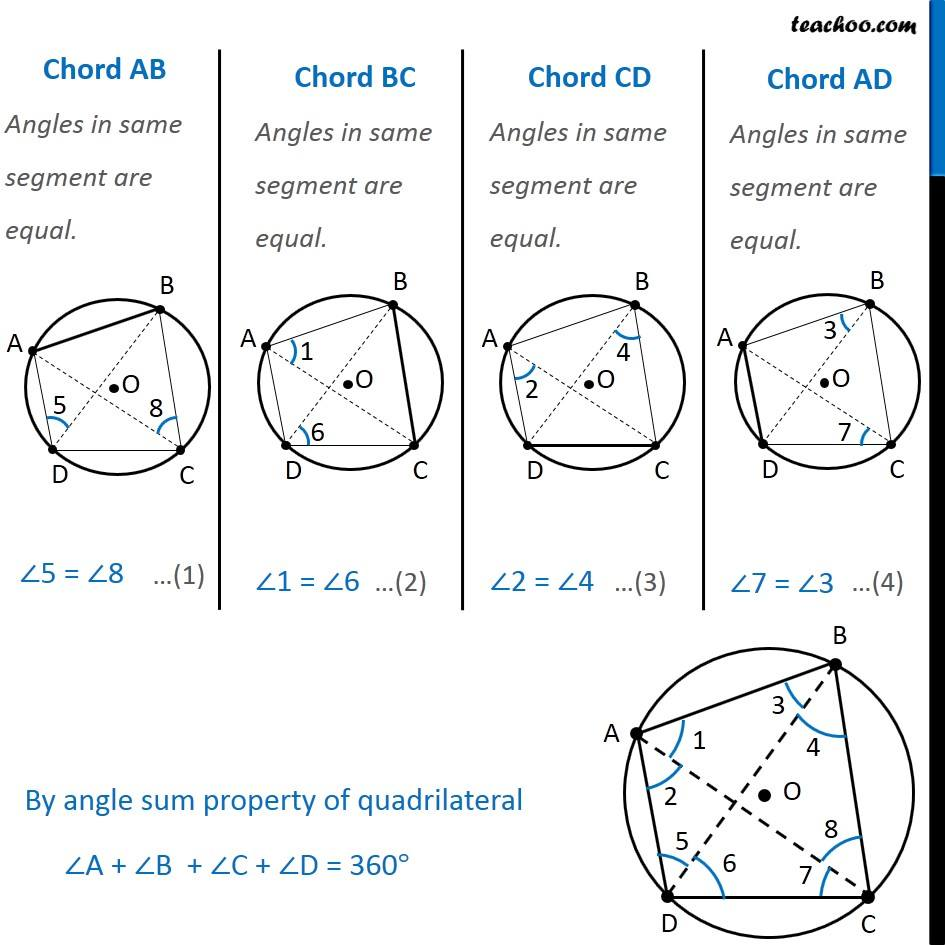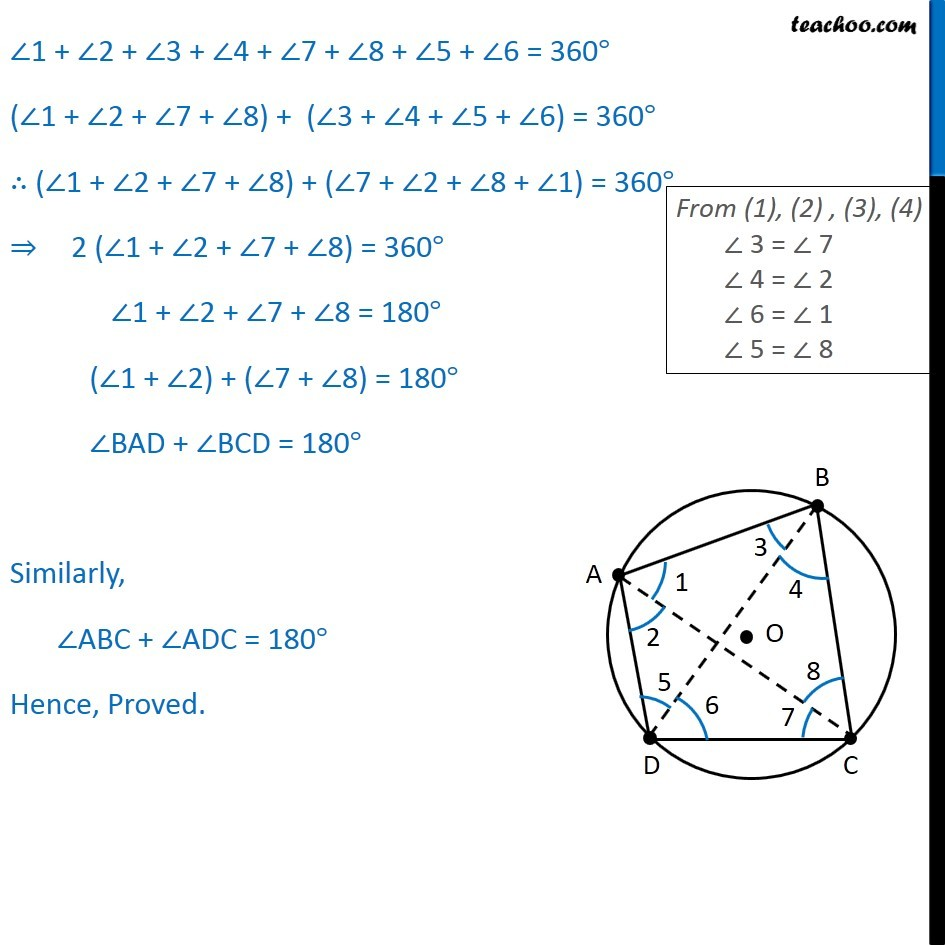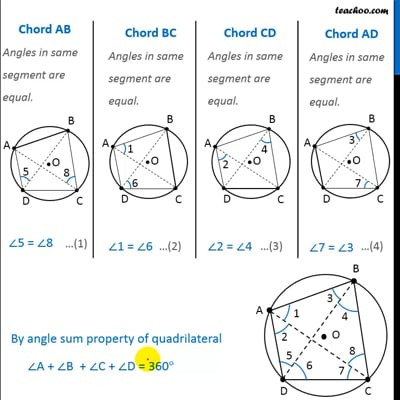Chapter 10 Class 9 Circles (Term 2)
Concept wiseThis video is only available for Teachoo black users

### Transcript

Theorem 10.11 The sum of either pair of opposite angles of a cyclic quadrilateral is 180°. Given : ABCD is a cyclic quadrilateral. of a circle with centre at O To Prove : ∠ BAD + ∠ BCD = 180° ∠ ABC + ∠ ADC = 180° Proof: Chord AB Angles in same segment are equal. ∠5 = ∠8 Chord BC Angles in same segment are equal. ∠1 = ∠6 Chord CD Angles in same segment are equal. ∠2 = ∠4 Chord AD Angles in same segment are equal. ∠7 = ∠3 ∠1 + ∠2 + ∠3 + ∠4 + ∠7 + ∠8 + ∠5 + ∠6 = 360° (∠1 + ∠2 + ∠7 + ∠8) + (∠3 + ∠4 + ∠5 + ∠6) = 360° ∴ (∠1 + ∠2 + ∠7 + ∠8) + (∠7 + ∠2 + ∠8 + ∠1) = 360° ⇒ 2 (∠1 + ∠2 + ∠7 + ∠8) = 360° ∠1 + ∠2 + ∠7 + ∠8 = 180° (∠1 + ∠2) + (∠7 + ∠8) = 180° ∠BAD + ∠BCD = 180° Similarly, ∠ABC + ∠ADC = 180° Hence, Proved. From (1), (2) , (3), (4) ∠ 3 = ∠ 7 ∠ 4 = ∠ 2 ∠ 6 = ∠ 1 ∠ 5 = ∠ 8